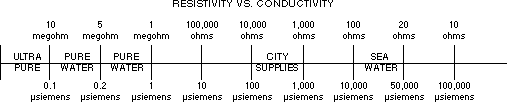# What is the relationship between Resistivity and Conductance?

## Document ID

Document ID TE2560

## Published Date

Published Date 04/10/2018
Question
What is the relationship between Resistivity and Conductance?
Summary
Relationship between resistivity and conductance
Resistivity is the inverse of Conductance.

Resistivity in units of ohms = the inverse of Conductivity in units of siemens

Example:  a million ohms = a millionth siemens

1,000,000 Ohms = 1 Megaohm = 1 Mohm = 1 x 10-6 siemens = 1 microsiemens = 1 μS

Here is a chart with Resistivity values on top and Conductance values on bottom.Source: Myron L Co